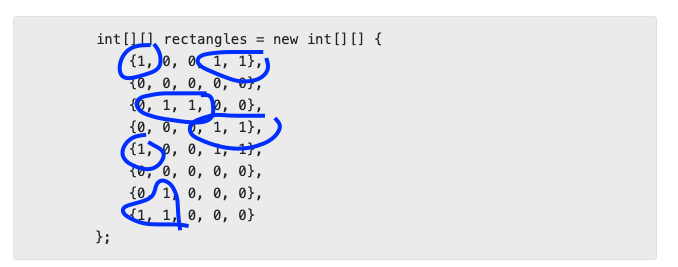Apr 23, 2019

# Algorithms How to Search a Graph with Depth First Search

Recently I’ve been reviewing some Algorithms, and was specifically looking at ways to search a Graph with Depth First Search (DFS). I thought it was kinda cool and wanted to write about it.

Many times in code challenges or academics you’ll be presented with a situation where you have an image or a map. The challenge will be to chart out some shape on the map, or identify how many individual images are presented etc.

DFS is perfect for this.

In the code I’m going to be sharing here, I’m using a 2D array of points to represent a map. The land on the map is represented with 1s and the water is represented with 0s. An example map would be the following:

``````int[][] rectangles = new int[][] {
{1, 0, 0, 1, 1},
{0, 0, 0, 0, 0},
{0, 1, 1, 0, 0},
{0, 0, 0, 1, 1},
{1, 0, 0, 1, 1},
{0, 0, 0, 0, 0},
{0, 1, 0, 0, 0},
{1, 1, 0, 0, 0}
};``````

Just to provide a better visual, I’ve circled the land in blue in the following screenshot:So the way that you would search this array is basically the following:

1. Walk through each element in the array
2. If land (1s) is found, use recursion to investigate all the cells around this land until you encounter 0s
3. All 1s that are encountered get marked out with 0s. This is so you can keep track of where you have been.
4. When you finish do something with either the coordinates or land that you have counted.

The following are two methods which take in a 2D array and then search the values. This is done in Java.

``````public static ArrayList<ArrayList<Integer>> searchLand(int[][] land) {

ArrayList<ArrayList<Integer>> output = new ArrayList<ArrayList<Integer>>();

// make a copy to do the work without modifying the original
int[][] land2 = land;

ArrayList<ArrayList<int[]>> coordinates = new ArrayList<ArrayList<int[]>>();

for(int row = 0; row < land2.length; row++) {
for(int col = 0; col < land2[row].length; col++) {
if(land2[row][col] == 1) {
ArrayList<int[]> foundCoordinates = new ArrayList<int[]>();
landDFS(land2, row, col, foundCoordinates);
}
}
}

System.out.println("found coordinates " + coordinates.size());
System.out.println("");

for(ArrayList<int[]> found: coordinates) {
for(int[] value: found){
System.out.println("row " + value + " col " + value);
}
System.out.println("");
System.out.println("");
}

return output;
}

public static void landDFS(int[][] land2, int row, int col, ArrayList<int[]> foundCoordinates) {
if(col < 0 || row < 0 || (row > land2.length - 1) || (col > land2[row].length - 1)) {
return;
}

if(land2[row][col] == 1){
land2[row][col] = 0;
// left
landDFS(land2, row, col - 1, foundCoordinates);
// right
landDFS(land2, row, col + 1, foundCoordinates);
// above
landDFS(land2, row + 1, col, foundCoordinates);
// below
landDFS(land2, row - 1, col, foundCoordinates);
}
}``````

If you use the example 2D array I provided above, the output should look similar to the following:

``````found coordinates 6

row 0 col 0

row 0 col 3
row 0 col 4

row 2 col 1
row 2 col 2

row 3 col 3
row 3 col 4
row 4 col 4
row 4 col 3

row 4 col 0

row 6 col 1
row 7 col 1
row 7 col 0``````

The reason that this is considered DFS is because it goes as far down as possible before moving onto the next cell. A Breadth First Search(BFS) version of this same algorithm would use a Queue to search the closest elements first before proceeding. The use of DFS here makes the most sense because the entire Graph needs to be searched. I’m going to be writing a future post on BFS which will cover the use cases for both.

Hope I’ve provided a good explanation of DFS, and how you can use it to search a graph.